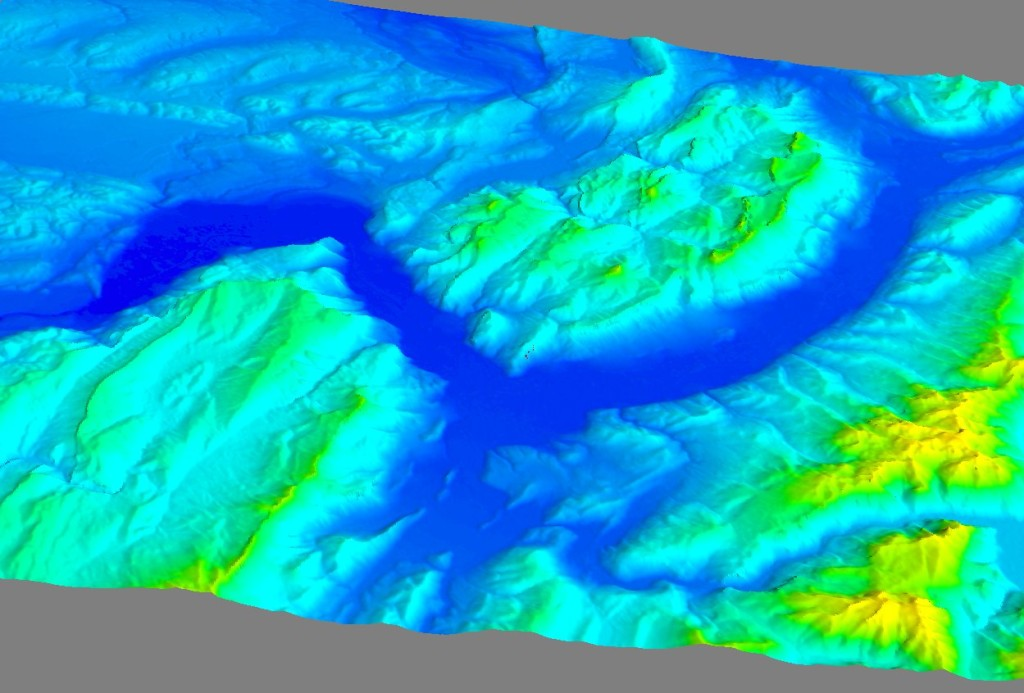# OpenStreetMap/SRTM 3D display

## What ?

I wanted to display OpenStreetMap hiking paths on 3D terrain to get a better idea of hiking routes.## How ?

I used python and some nice libraries (Mayavi mlab, gdal, Numpy, and Osm Python API) to learn how to do this. This is pretty much a work-in-(very slow)-progress. I used many ressources to make this (see bookmarks).

I had to make a custom version of OSM Python API to add a basic http proxy support.

I selected a hiking trail near Grenoble in OpenStreetMap, clicked on “edit” and got the ID of the path. The relevant SRTM file was downloaded from here to get the 3D elevation data.

Next steps should be :

• download and render more than just one hiking path (using OsmApi, that should be easy enough)
• get better elevation data (hard…, seems IGN for France is not free to use at 25m resolution)
• get GPS tracks from OpenStreetMap to get better elevation data on small surfaces (needs an update to Python OSM API)
• display textures from satellite imaging or equivalent
• display textures from rendered OSM tiles (including forest/rock/grass…)

Here is the code (comments should be enough to explain)

```# -*- coding: utf-8 -*-

from enthought.mayavi import mlab
import numpy
from osgeo import gdal, osr
import OsmApi
import math

# Read elevation data file SRTM
# Read GeoTiff and get coordinates
ds = gdal.Open('/home/.../OpenStreetMap/SRTM/srtm_38_03.tif')

width = ds.RasterXSize
height = ds.RasterYSize
gt = ds.GetGeoTransform()
minx = gt
maxx = gt + width*gt + height*gt
miny = gt + width*gt + height*gt
maxy = gt

band = ds.GetRasterBand(1)

# Grenoble zone to display = 400->1400, 5300->6000 pixels
zoneXmin = 400
zoneXsize = 1000
zoneYmin = 5300
zoneYsize = 695
data = band.ReadAsArray(400, 5300, 1000, 695).astype(numpy.float)

def degrees2metersLongX(latitude, longitudeSpan):
""" latitude (in degrees) is used to convert a longitude angle to a distance in meters """

def degrees2metersLatY(latitudeSpan):
""" Convert a latitude angle span to a distance in meters """

def degrees2meters(longitude, latitude):
return (degrees2metersLongX(latitude, longitude), degrees2metersLatY(latitude))

# CreateX,Y coordinates for data array (contains Z in meters)
linex = numpy.arange(minx+zoneXmin*gt, minx+(zoneXmin+zoneXsize)*gt, gt)[0:zoneXsize]
arrayx = numpy.tile(linex, (len(data), 1)).transpose()

lineydegrees = numpy.arange( maxy+zoneYmin*gt, maxy+(zoneYmin+zoneYsize)*gt, gt)[0:zoneYsize]
liney = numpy.array([degrees2metersLatY(j) for j in lineydegrees])
arrayy = numpy.tile(liney, (len(data), 1))

# Recompute arrayx positions in meters
for x, y in numpy.ndindex(arrayx.shape):
arrayx[x,y] = degrees2metersLongX(lineydegrees[y], arrayx[x,y])

zscale = 1

# Display 3D surface
mlab.mesh(arrayx, arrayy, data.transpose())

# OpenStreetMap
osm = OsmApi.OsmApi(proxy="******", proxyport = 3128)

# Bec du Corbeau hiking path : http://www.openstreetmap.org/browse/way/47189761 --> ID 47189761
way = osm.WayGet(47189761)
nodesId = way[u'nd']
nodes = osm.NodesGet(nodesId)
allX = []
allY = []
allZ = []

for nid in nodes:
n=nodes[nid]
(x,y) = degrees2meters(n['lon'], n['lat'])
allX.append(x)
allY.append(y)
zz = data.transpose()[int(round((n['lon'] - (minx+zoneXmin*gt)) / gt)), int(round((n['lat'] - (maxy+zoneYmin*gt)) / gt))]
allZ.append(zz+30.0) # We display the path 30m over the surface for it to be visible

# Display path nodes as spheres
mlab.points3d(allX,allY,allZ, color=(1,0,0), mode='sphere', scale_factor=30)
# Displaying the line does not work as nodes are not listed in the correct order
# mlab.plot3d(allX,allY,allZ, color=(1,1,0), line_width=15.0) # representation='surface' 'wireframe' 'points'

# Display a black dot on Grenoble's coordinates
mlab.points3d([5.717*degrees2meters], [45.183*degrees2meters],, color=(0,0,0), mode='sphere', scale_factor=50)```

## Result

• The three-valleys “Y” of Grenoble• A zoomed-in image to see the path